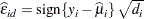### Residual Analysis

The model errors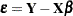are unobservable. Yet important features of the statistical model are connected to them, such as the distribution of the data, the correlation among observations, and the constancy of variance. It is customary to diagnose and investigate features of the model errors through the fitted residuals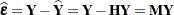. These residuals are projections of the data onto the null space ofand are also referred to as the "raw" residuals to contrast them with other forms of residuals that are transformations of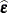. For the classical linear model, the statistical properties ofare affected by the features of that projection and can be summarized as follows: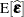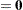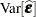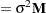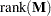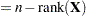Furthermore, if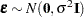, then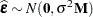.

Because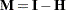, and the "hat" matrix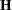satisfies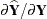, the hat matrix is also the leverage matrix of the model. If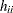denotes theth diagonal element of(the leverage of observation), then the leverages are bounded in a model with intercept,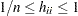. Consequently, the variance of a raw residual is less than that of an observation: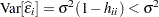. In applications where the variability of the data is estimated from fitted residuals, the estimate is invariably biased low. An example is the computation of an empirical semivariogram based on fitted (detrended) residuals.

More important, the diagonal entries ofare not necessarily identical; the residuals are heteroscedastic. The "hat" matrix is also not a diagonal matrix; the residuals are correlated. In summary, the only property that the fitted residualsshare with the model errors is a zero mean. It is thus commonplace to use transformations of the fitted residuals for diagnostic purposes.

#### Raw and Studentized Residuals

A standardized residual is a raw residual that is divided by its standard deviation: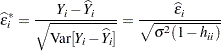Because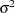is unknown, residual standardization is usually not practical. A studentized residual is a raw residual that is divided by its estimated standard deviation. If the estimate of the standard deviation is based on the same data that were used in fitting the model, the residual is also called an internally studentized residual: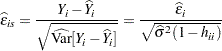If the estimate of the residual’s variance does not involve theth observation, it is called an externally studentized residual. Suppose that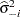denotes the estimate of the residual variance obtained without theth observation; then the externally studentized residual is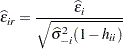#### Scaled Residuals

A scaled residual is simply a raw residual divided by a scalar quantity that is not an estimate of the variance of the residual. For example, residuals divided by the standard deviation of the response variable are scaled and referred to as Pearson or Pearson-type residuals: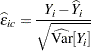In generalized linear models, where the variance of an observation is a function of the meanand possibly of an extra scale parameter,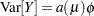, the Pearson residual is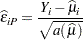because the sum of the squared Pearson residuals equals the Pearson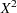statistic: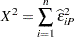When the scale parameter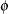participates in the scaling, the residual is also referred to as a Pearson-type residual: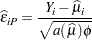#### Other Residuals

You might encounter other residuals in SAS/STAT software. A "leave-one-out" residual is the difference between the observed value and the residual obtained from fitting a model in which the observation in question did not participate. If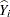is the predicted value of theth observation and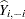is the predicted value if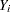is removed from the analysis, then the "leave-one-out" residual is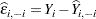Since the sum of the squared "leave-one-out" residuals is the PRESS statistic (prediction sum of squares; Allen 1974),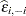is also called the PRESS residual. The concept of the PRESS residual can be generalized if the deletion residual can be based on the removal of sets of observations. In the classical linear model, the PRESS residual for case deletion has a particularly simple form: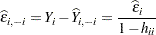That is, the PRESS residual is simply a scaled form of the raw residual, where the scaling factor is a function of the leverage of the observation.

When data are correlated,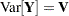, you can scale the vector of residuals rather than scale each residual separately. This takes the covariances among the observations into account. This form of scaling is accomplished by forming the Cholesky root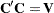, where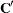is a lower-triangular matrix. Then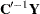is a vector of uncorrelated variables with unit variance. The Cholesky residuals in the model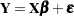are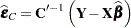In generalized linear models, the fit of a model can be measured by the scaled deviance statistic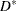. It measures the difference between the log likelihood under the model and the maximum log likelihood that is achievable. In models with a scale parameter, the deviance is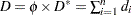. The deviance residuals are the signed square roots of the contributions to the deviance statistic: